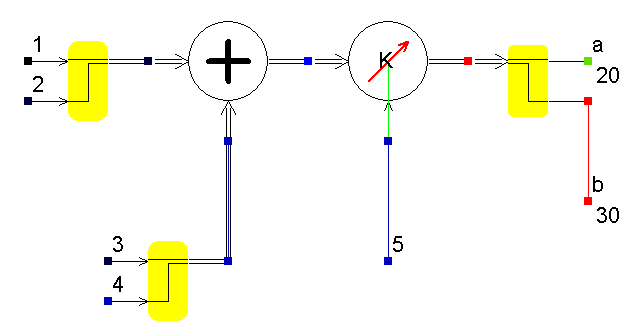• Introduction
• What is in this manual
• What is Caspoc
• User interface
• Introduction
• Starting
• Simulation
• Editing
• Viewing and printing
• Getting Started
• Basic editing
• Simulation in the time domain
• Basic User Interface Topics
• Editing
• Simulation
• Viewing
• Library
• Reports
• Project management
• Circuit and Block Diagram Components
• Introduction
• Cscript and user defined functions
• Component parameters
• Modeling Topics
• Introduction
• Power Electronics
• Semiconductors
• Electrical Machines
• Electrical drives
• Power Systems
• Mechanical Systems
• Thermal Systems
• Magnetic Circuits
• Green Energy
• Coupling to FEM
• Experimenter
• Analog hardware description language
• Embedded C code Export
• Coupling to Spice
• Small Signal Analysis
• Matlab coupling
• Tips and tricks
• Appendices

## Editing the Space vector format.

The difference between the space vector components and the scalar block-diagram components is that the former requires two signals to be passed from component to component.
Therefore the space vector components have multiplexed input and outputs that you can recognize by the double lines in the connections, as shown in the figure below:The double lines are drawn automatically by the program once you start the simulation. When you start the simulation, Caspoc first sorts out which lines are representing space vectors and replaces the single lines with double lines. The space vector format is always defined by the output from a component. All components that you select in the Advanced Electrical Drives library from the /Vector sections are space vector models

Numerical value
The numerical value directly measured by the mouse pointer at the node is the amplitude of the space vector.

Interface between Scalar and Vector components
The interface between the scalar and space vector format is given by the blocks Vector2Scalar and Scalar2Vector from the section "Components/Library/AdvancedElectricalDrives/Interface".

Color of the connections
In the book Fundamentals of Electrical Drives the stator, rotor an mechanical sections have different colors. In caspoc you can color the components and connections, by selecting the components and setting the background color in the menu Insert/Color Selected Components

• Select all components and connections by drawing a ruber band around them.(Start with the left mouse button donw and end the selection by releasing the left mouse button)
• Select background color from the menu Insert/Color Selected Components
Inside the component dialog box you can define the components fill and frame color. An exception is the IRTF block which has predefined input and output colors.